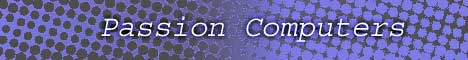Home  | Computers  | Networking  | Programming  | Electronics  | How to   | Help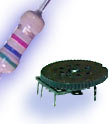Jump to Carbon film resistors Chip resistors Network resistors Wire wound resistors Variable resistors Circuit combinations Ohms law Finding unknown values Related The inductor The Transformer Making circuit boards

 Introduction
 Below are some of the most basic and most abundant resistor patterns you will see in the electronics field. You will see these patterns in many circuits for two main reasons and the first being that it may be difficult and/or expensive to create a resistive level with just one resistor. The other reason being that in many circuits a component such as the variable resistor will be in parallel with a capacitor or an inductor to produce such circuits as bass and tone control for radios. The possibilities are endless and you will see these same circuits many times in the field of electronics.
 Finding resistance
There are basically three different types of circuits that the resistor can be in.

Series
In this situation, to find the total resistance is to basically add up all the resistor values.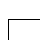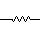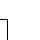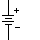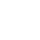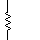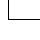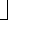R1 + R2 + R3 = total resistance " Rt "
 I have found out my resistance, now how do I use it in a circuit?

Parallel
To find the total resistance of a parallel circuit you would divide one by each of the resistors. Next add them up and once again divide one by your final answer. For example, if you have a 25ohm, 30ohm, and 18ohm set of resistors you would do the following.
1/25 + 1/30 + 1 /18 = .04 + .0333 + .0555 = 0.1288
1/0.1288 =7.76 ohms

The total resistance of your series circuit is 7.76, notice that the sum of all the resistors are less then R1, R2, or R3.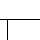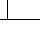1   +  1   + 1       1
R1   R2     R3 = Rt

 I have found out my resistance, now how do I use it in a circuit?

Series-parallel circuit
In the following example we have a circuit that is a combination of both series and parallel circuits. The equation for solving this circuit is to first find the values of your parallel circuit. The next step is to treat the total resistive value of your parallel circuit as one resistor. With that in mind you will add the total resistance in your parallel circuit with the remaining series resistors to get your final value.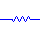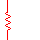1   + 1   = 1
R2   R3   Rt
+ R1 + R4
 I have found out my resistance, now how do I use it in a circuit?
 Page    1 2 3 Next

Home | Contact us | Our History | Link to us | Relations
A Passion Production ©opyright 2000